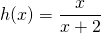Chapter 11: Functions

# 11.3 Inverse Functions

When working with mathematical functions, it sometimes becomes useful to undo what the original function does. To do this, you need to find the inverse of the function. This feature is commonly used in exponents and logarithms and in trigonometry.

In this topic, you will be looking at functions and seeing if they can be inverses of themselves. The notation used for this procedure is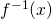is the inverse of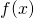. In practice, this works as follows: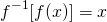This is a very useful tool used many times over in math. If there are two functionsand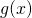that are inverses of each other (if their composites “undo” each other’s function), their composite functions look like: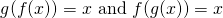Example 11.3.1

Are the functions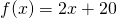and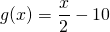inverses of each other?

Test if either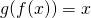or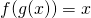: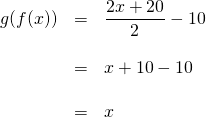These two functions are inverses of each other. If you had tested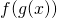, you would have gotten the same result,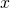.

Example 11.3.2

Are the functions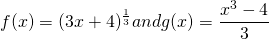inverses of each other?

Test if eitheror.

For this problem, it would be easier to work with, since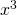will cancel out the radical in the.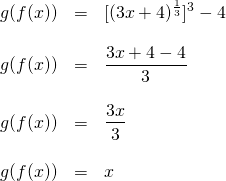These functions are inverses of each other.

One of the strategies that is used to find the inverse of another function involves the substitution of theand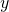variables of an equation. This is shown in the next few examples.

Example 11.3.3

Find the inverse function of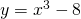.

The inverse function is found by substitutingfor allvalues andfor allvalues in the original equation and then isolating for.

From the equation, you now get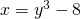.

Isolating foryields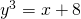, which simplifies to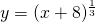.

These equations can be also written as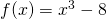and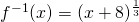.

Example 11.3.4

Find the inverse function of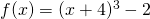.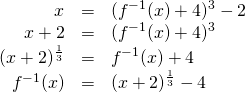# Questions

State if the given functions are inverses.

1.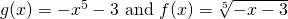2.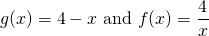3.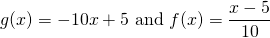4.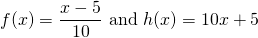5.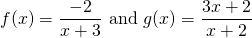6.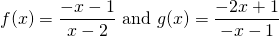For questions 7 to 22, find the inverse of each function.

1.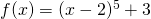2.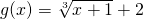3.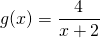4.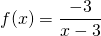5.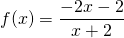6.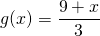7.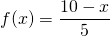8.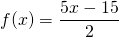9.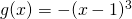10.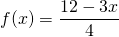11.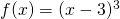12.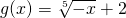13.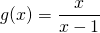14.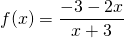15.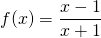16.